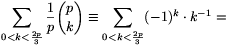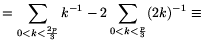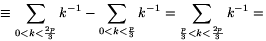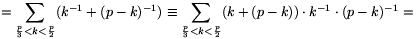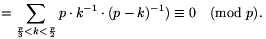Mathematical and Physical Journal
for High Schools
Issued by the MATFUND Foundation
 Already signed up? New to KöMaL?# Solutions for problems "A" in September, 2001

In this page only the sketch of the solutions are published; in some cases only the final results. To achieve the maximum score in the competition more detailed solutions needed.

A. 269. A round hole is to be completely covered with two square boards. The sides of the squares are 1 metre. In what interval may the diameter of the hole vary?

Solution. It is easy to see that a hole of radius $\displaystyle 2-\sqrt2$ (and all smaller holes) can be covered by two unit squares, see Figure 1.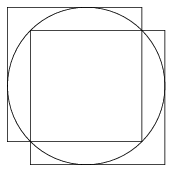Figure 1

We will prove that if a unit square covers at least half of the perimeter of a circle of radius r, then $\displaystyle r\le2-\sqrt2$. This implies that the diameter of the hole cannot exceed $\displaystyle 4-2\sqrt2\approx1.17$ metre.

Let K be a circle of radius r, and N be a unit square, which covers at least the half of K. If r$\displaystyle ge$1/2, then N can be translated such that each side of N or its extension has at least one common point with K and the covered part of the curve is increasing, see Figure 2.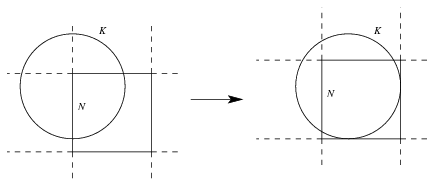Figure 2

So, it can be assumed that r>1/2 and (the extension of) each side of N has a common point with K.

First, consider the case when a vertex of N is inside K. Let the vertices of N be A, B, C, D and suppose that A is inside K. Denote the intersecion of K and the half-lines AB and AD by P and Q, respectively. (Figure 3.)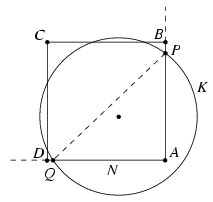Figure 3

Because PAQ$\displaystyle angle$=90o, the centre of K and vertex A are on the same side of line PQ. This implies that the uncovered arc PQ is longer than the half of K. This is a contradiction, thus this case is impossible.

We are left to deal the case when there are no vertices of N inside K and each side or its extension has a common point with K. It is easy to check that these common points cannot be on the extension of the sides of N; they must lie on the perimeter of N.

Denote by $\displaystyle alpha$, $\displaystyle beta$, $\displaystyle gamma$ and $\displaystyle delta$ the angles drawn in Figure 4.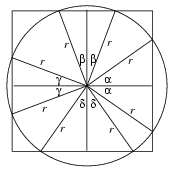Figure 4

The total of the uncovered arcs is at most the half of K, so $\displaystyle alpha$+$\displaystyle beta$+$\displaystyle gamma$+$\displaystyle delta$90o. By symmetry, it can be assumed that+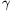45o.

As can be read from the Figure, r(cos+cos)=r(cos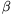+cos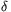)=1. The concavity of the cosine function yields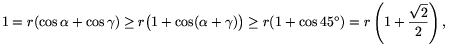and hence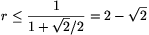.

Thus, the radius of the hole can be at most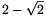; its diameter can be at most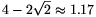metre.

A. 270. Prove that if a, b, c, d are positive numbers then

 (1)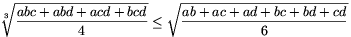Solution 1. Define the folowing symmetric expressions of a, b, c, d:

S33=a3b3+a3c3+a3d3+b3c3+b3d3+c3d3;

S321=a3b2c+a3b2d+...+bc2d3;

S3111=a3bcd+ab3cd+abc3d+abcd3;

S222=a2b2c2+a2b2d2+a2c2d2+b2c2d2;

S2211=a2b2cd+a2bc2d+a2bcd2+ab2c2d+ab2cd2+abc2d2.

Taking the 6th power of (1), we obtain

 (2) 2S33+6S321+12S311115S222+24S2211.

By the A.M.-G.M. inequality

2S33=(a3b3+a3c3+b3c3)+(a3b3+a3d3+b3d3)+

+(a3c3+a3d3+c3d3)+(b3c3+b3d3+c3d3)3a2b2c2+3a2b2d2+3a2c2d2+3b2c2d2=3S222.

We similarly obtain that S3216S222, S3214S2211 and 3S31112S2211. Thus

2S33+6S321+12S3111=2S33+2.S321+4.S321+4.3S31113S222+2.6S222+4.4S2211+4.2S2211=15S222+24S2211.

Solution 2. The polynomial f(x)=(x-a)(x-b)(x-c)(x-d) has four positive (not necessariliy different) roots. Thus, its derivative has three positive roots; denote them by u, v and w.

By Viete's formulae,

f'(x)=4x3-3(a+b+c+d)x2+

=4(x3-(u+v+w)x2+(uv+uw+vw)x-uvw).

Thus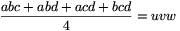and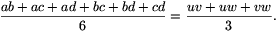Substituting these in (1), it becomes the inequality between the arithmetic and geometric means of uv, uw and vw.

A. 271. Prove that for any prime, p5, the number

 (1)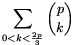is divisible by p2.

Vojtech Jarnik Mathematics Competition, Ostrava, 2001

Solution. Each term of (1) is divisible by p, so our goal is to prove that

 (2)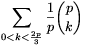is divisible by p.

For an arbitrary integer 1x<p, let x-1 be the multiplicative inverse of x modulo p.

For all 0<k<p,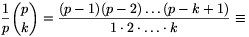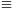(-1).(-2).....(-k+1).1-1.2-1.....k-1(-1)k.k-1 (mod p),

and